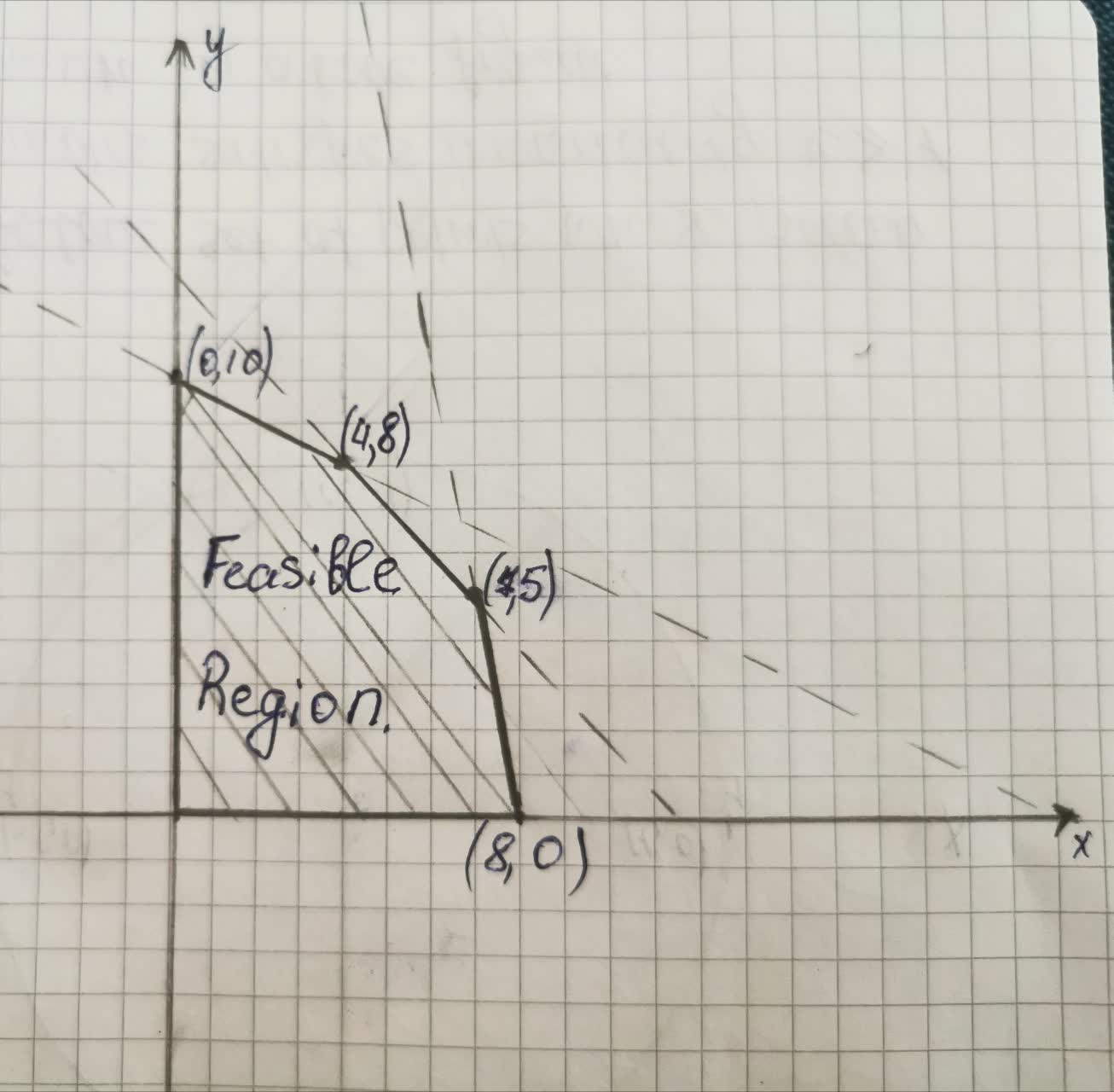# Tara dog bakery makes dog treats ultimate peanut butter and cheesy butter.tabita57i 2021-08-31 Answered
Tara dog bakery makes dog treats ultimate peanut butter and cheesy butter.
Ultimate peanut butter requires 2 hours of kitchen time 5 pounds of peanut butter and 1 worker each batch of chessy butter requires 4 hours of kitchen time 1 pound peanut butter 1 worker the bakery has 40 kitchen hours each week 40 pounds of peanut butter and 12 workers if each batch of ultimate peanutbutter brings revenue of 20 dollars and each batch of cheesy butter brings revenue of 30 dollars in.
How many batches of each flavor should the bakery make to maximize revenue show all equations graphing of feasible reigons corner points identified and calculations to show maximum revenue combinations.

• Questions are typically answered in as fast as 30 minutes

### Plainmath recommends

• Get a detailed answer even on the hardest topics.
• Ask an expert for a step-by-step guidance to learn to do it yourself.Bentley Leach

Let x be the number of batches of ultimate peanut butter.
Let y be the number of batches of cheesy butter.
Here it is given that ultimate peanut butter requires 2 hours of kitchen time 5 pounds of peanut butter and 1 worker each batch and chessy butter requires 4 hours of kitchen time 1 pound peanut butter 1 worker for each batch.
In total the bakery has 40 kitchen hours each week 40 pounds of peanut butter and 12 workers.
Therefore, $$\displaystyle{2}{x}+{4}{y}\leq{40}$$
$$\displaystyle{5}{x}+{y}\leq{40}$$
$$\displaystyle{x}+{y}\leq{12}$$
Now, each batch of ultimate peanutbutter brings revenue of 20 dollars and each batch of cheesy butter brings revenue of 30 dollars.
We have to find maximum revenue. Therefore, we have to maximize
$$\displaystyle{20}{x}+{30}{y}$$
Maximize $$\displaystyle{z}={20}{x}+{30}{y}$$
$$\displaystyle{2}{x}+{4}{y}\leq{40}$$
$$\displaystyle{5}{x}+{y}\leq{40}$$
$$\displaystyle{x}+{y}\leq{12}$$Corner Points are ($$\displaystyle{\left({0},{0}\right)},{\left({8},{0}\right)},{\left({7},{5}\right)},{\left({4},{8}\right)}{\quad\text{and}\quad}{\left({0},{10}\right)}$$.
We have to find the maximum value of $$z=20x+30y$$
Therefore,
At $$(0,0), z= 0+0 =0$$
At $$(8,0), z= 160+0 =160.$$
At $$(7,5), z=140+150 = 290.$$
At $$(4,8), z=80+240 = 320.$$
At $$(0,10), z=0+300 = 300$$.
Therefore, Maximum $$z=320$$ at $$x=4$$ and $$y=8$$.